# Brain Tumor Segmentation Using Fuzzy C-Means Clustering

This examples shows how to segment brain tumors from 3-D medical images using fuzzy c-means (FCM) clustering algorithms.

### Brain Tumor Segmentation

Automatic detection of brain tumors using medical images plays a vital role in the diagnosis process. High resolution magnetic resonance (MR) images are a popular choice to diagnose brain tumors by identifying abnormal brain tissue. MR image segmentation helps to partition brain tissue into multiple regions, based on characteristics like intensity, color, and texture. One segmentation approach is image clustering, which is a form of unsupervised classification that groups similar data (pixels) together by comparing the distance of each data point to different cluster centers.

Different brain tissues, including cerebrospinal fluid, white matter, and gray matter, are not well-defined in MR intensity images. As a result, the edges between regions blend together, as shown in the following MR image slice.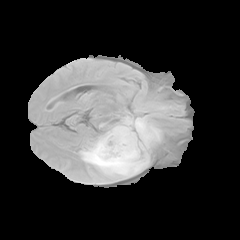Fuzzy clustering algorithms take inspiration from fuzzy inference systems and provide each data point different levels of membership in each cluster. As a result, fuzzy clustering algorithms are commonly used for brain tumor segmentation to handle the overlapping cluster representation of brain tissues in MR images.

### Fuzzy C-Means Clustering for Tumor Segmentation

The fuzzy c-means algorithm  is a popular clustering method that finds multiple cluster membership values of a data point. Extensions of the classical FCM algorithm generally depend on the type of distance metric calculated between data points and cluster centers. This example demonstrates brain tumor segmentation using the classical FCM method, which uses a Euclidean distance metric, and Gustafson-Kessel (GK) extension, which uses a Mahalanobis distance metric.

The segmentation process includes the following steps:

1. Prepare feature vectors from the training and testing data.

2. Cluster the training data using specified distance metric.

3. Identify the ientified cluster that represents the tumor.

4. Use the identified cluster centers to identify tumors in the testing data.

After performing these steps using both distance metrics, you can compare the clustering results.

To run this example using previously saved clustering results, set `runFCM` to `false`. To run the clustering process, set this value to `true`.

`runFCM =false;`

This example uses the sample BraTS data set , which contains 4-D volumes, each representing a stack of 3-D volumetric scans. Each 4-D volume has size 240-by-240-by-152-by-4, where the first three dimensions correspond to the height, width, and depth of a 3-D volumetric image. The fourth dimension represents different scan modalities. For this example, you use only data from the first scan modality.

Download two volumes, one for training and one for testing, and test labels from the sample data set for this example.

```imageDir = fullfile(tempdir,"BraTS"); filename = matlab.internal.examples.downloadSupportFile(... "vision","data/sampleBraTSTestSetValid.tar.gz"); untar(filename,imageDir); trainDataFileName = fullfile(imageDir,... "sampleBraTSTestSetValid\imagesTest\BraTS447.mat"); testDataFileName = fullfile(imageDir,... "sampleBraTSTestSetValid\imagesTest\BraTS463.mat"); testLabelFileName = fullfile(imageDir,... "sampleBraTSTestSetValid\labelsTest\BraTS463.mat");```

### Create Training and Testing Data

First reshape the first scan modality from the training data from a 240-by-240-by-152-by-1 matrix to a 57,600-by-152 matrix, where each column represents a vertical slice of the 3-D volume data set.

```orgTrainData = load(trainDataFileName); [r,c,n,~] = size(orgTrainData.cropVol); trainingData = reshape(orgTrainData.cropVol(:,:,:,1),[r*c n]);```

Next, create feature vectors from the training data to capture spatial patterns in the data. Use a moving window over each pixel of the training data and flatten out the subimage captured under the window as a row feature vector. Larger window sizes can result in overlaps of different patterns in the same subimage.

For this example, use a 3-by-3 window size, which produces a nine-element feature vector for each data point. Create a feature matrix with nine columns for the features using the `createMovingWindowFeatures` helper function included at the end of this example. Each row of the matrix corresponds to one data point.

```kDim = [3 3]; trainFeatures = createMovingWindowFeatures(trainingData,kDim);```

Similarly, reshape the testing data set to a 57,600-by-152 matrix.

```orgTestData = load(testDataFileName); testData = reshape(orgTestData.cropVol(:,:,:,1),[r*c n]);```

Create test feature vectors from the test data.

`testFeatures = createMovingWindowFeatures(testData,kDim);`

Load labeled data for the testing images. This data indicates which pixels in the testing data represent tumors.

```orgLabel = load(testLabelFileName); refLabel = orgLabel.cropLabel;```

Identify labeled tumor pixel indices for each image and set a flag if an image contains tumor pixels.

```refTumor = cell(1,n); % Tumor pixel ids refHasTumor = false(1,n); for id = 1:n refTumor{id} = find(refLabel(:,:,id)==1); refHasTumor(id) = ~isempty(refTumor{id}); end```

### Segment Image

Specify Training Options

Configure the clustering options to specify 15 clusters and a maximum of 10 iterations.

```numClusters = 15; options = fcmOptions; options.NumClusters = numClusters; options.MaxNumIteration = 10;```

Cluster Data Using Euclidean Distance

To use the classical FCM algorithm, set the `DistanceMetric` option to `"euclidean"`.

`options.DistanceMetric = "euclidean";`

Cluster the training data using the `fcm` function. For consistent results, initialize the random number generator before calling `fcm`.

```if runFCM rng("default") %#ok<*UNRCH> ecCenters = fcm(trainFeatures,options); else load("fcmBrainTumorSegmentationResults.mat","ecCenters"); end```

Find the distance from each data point in the testing data to each calculated cluster center.

`ecDist = findDistance(ecCenters,testFeatures);`

For each data point, find the index of the closest cluster center.

`[~,ecLabel] = min(ecDist',[],2); %#ok<UDIM>`

Reshape the cluster center index array to match the original image dimensions. `ecLabel` contains the cluster label for each image pixel in the testing data.

```ecLabel = reshape(ecLabel,n,r*c)'; ecLabel = reshape(ecLabel,[r c n]);```

Identify tumor pixels in each test image using the `segmentTumor` helper function included at the end of this example. To use this function, you specify the tumor cluster index. You can use manual inspection of the training data to identify the tumor cluster, which here is the third cluster returned. The function labels the tumor pixels in the image, calculates the number of false positive pixels, and returns a logical value indicating whether the image contains a tumor. You can separately tune a fuzzy inference system (FIS) to automatically detect tumors from MR images.

```ecTumor = zeros(r,c,n); ecHasTumor = zeros(1,n); ecNumFalsePos = zeros(1,n); tumorCluster = 3; for id=1:n [ecHasTumor(id),ecNumFalsePos(id),ecTumor(:,:,id)] = ... segmentTumor(ecLabel(:,:,id),refTumor{id},tumorCluster); end```

View four images for one test case: original test image, segmented image, tumor pixels detected in the test image, and labeled image showing the ground truth of tumor pixels.

```figure refId = round(n/2); subplot(2,2,1) imshow(orgTestData.cropVol(:,:,refId,1)) xlabel("Test Image") subplot(2,2,2) imshow(ecLabel(:,:,refId)/numClusters) xlabel("Segmented Image") subplot(2,2,3) imshow(ecTumor(:,:,refId)) xlabel("Tumor Detection") subplot(2,2,4) imshow(refLabel(:,:,refId)) xlabel("Labeled image")```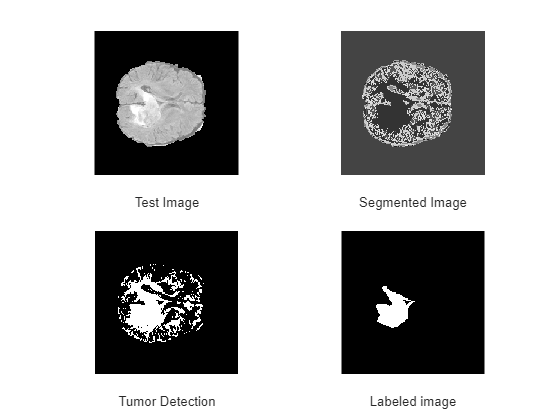Cluster Data Using Mahalanobis Distance

To use the GK clustering method, set the `DistanceMetric` option to `"mahalanobis"`.

`options.DistanceMetric = "mahalanobis";`

Cluster the training data.

```if runFCM rng("default") mnCenters = fcm(trainFeatures, options); else load("fcmBrainTumorSegmentationResults.mat","mnCenters"); end```

Find the distance from each data point in the testing data to each calculated cluster center and determine the closest cluster center for each image pixel.

```mnDist = findDistance(mnCenters,testFeatures); [~,mnLabel] = min(mnDist',[],2); %#ok<UDIM> mnLabel = reshape(mnLabel,n,r*c)'; mnLabel = reshape(mnLabel,[r c n]);```

Identify tumor pixels in each test image and determine the number of false positives and whether the image contains a tumor. TAs before, you can use manual inspection to identify the tumor cluster in the training data. Here, this cluster is the 12th one returned.

```mnTumor = zeros(r,c,n); mnHasTumor = zeros(1,n); mnNumFalsePos = zeros(1,n); tumorCluster = 12; for id=1:n [mnHasTumor(id),mnNumFalsePos(id),mnTumor(:,:,id)] = ... segmentTumor(mnLabel(:,:,id),refTumor{id},tumorCluster); end```

As with the Euclidean clustering, create four images for one test case showing the segmentation and ground truth.

```figure subplot(2,2,1) imshow(orgTestData.cropVol(:,:,refId,1)) xlabel("Test Image") subplot(2,2,2) imshow(mnLabel(:,:,refId)/numClusters) xlabel("Segmented Image") subplot(2,2,3) imshow(mnTumor(:,:,refId)) xlabel("Tumor Detection") subplot(2,2,4) imshow(refLabel(:,:,refId)) xlabel("Labeled Image")```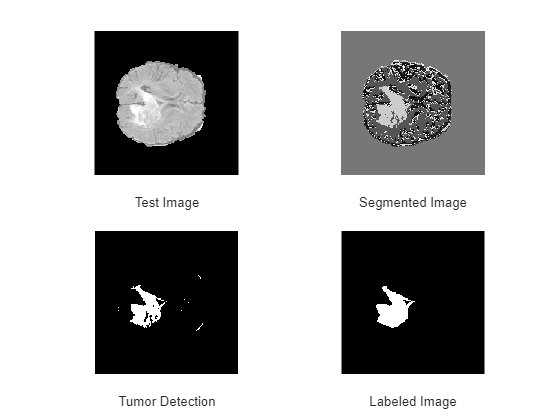### Compare Results for Different Distance Metrics

Visually compare the tumor-detection results for a single test image. From left to right, the plots show the tumor pixels for the ground truth labels, the Euclidean labels, and the Mahalanobis labels. The clustering using the Mahalanobis distance more closely matches the ground truth labels.

```figure title("Tumor Pixels") subplot(1,3,1) imshow(refLabel(:,:,refId)) xlabel("Ground Truth") subplot(1,3,2) imshow(ecTumor(:,:,refId)) xlabel("Euclidean Distance") subplot(1,3,3) imshow(mnTumor(:,:,refId)) xlabel("Mahalanobis Distance")```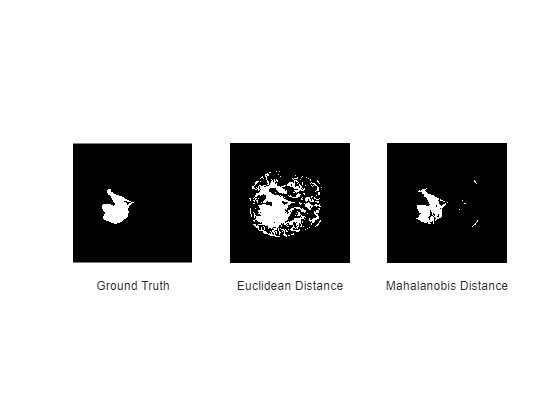The Mahalanobis metric provides fewer false positive pixels compared to the classical approach using a Euclidean distance metric.

Plot the number of pixel-level false positives across all images for both distance metrics.

```figure bar(ecNumFalsePos) hold on bar(mnNumFalsePos,"EdgeAlpha",0.75,"FaceAlpha",0.75); xlabel("Image sequence") ylabel("Number of pixels") hold off title("False Positives") legend(["Euclidean" "Mahalanobis"])```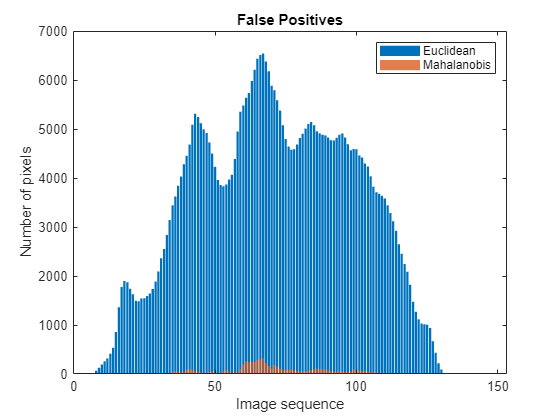Using the Mahalanobis distance metric produces fewer false positives for all images compared to the Euclidean distance metric.

You can also compare the tumor-detection performance for the two clustering methods. To do so, calculate true positive, true negative, false positive, and false negative values according to the tumor detection results using the distance metrics.

```depth = size(refLabel,3); ecTruePositive = length(find(refHasTumor & ecHasTumor)); ecFalseNegative = length(find(refHasTumor & ~ecHasTumor)); ecFalsePositive = length(find(~refHasTumor & ecHasTumor)); mnTruePositive = length(find(refHasTumor & mnHasTumor)); mnFalseNegative = length(find(refHasTumor & ~mnHasTumor)); mnFalsePositive = length(find(~refHasTumor & mnHasTumor)); truePositive = [ecTruePositive;mnTruePositive]; falsePositive = [ecFalsePositive;mnFalsePositive]; falseNegative = [ecFalseNegative;mnFalseNegative]; trueNegative = depth - (truePositive+falsePositive+falseNegative); tumorDetectionResults = table( ... truePositive,trueNegative,falsePositive,falseNegative, ... VariableNames=["True Pos" "True Neg" "False Pos" "False Neg"], ... RowNames=["Euclidean Distance" "Mahalanobis Distance"] ... ); disp(tumorDetectionResults)```
``` True Pos True Neg False Pos False Neg ________ ________ _________ _________ Euclidean Distance 80 26 46 0 Mahalanobis Distance 79 36 36 1 ```

The true positive values are similar for both FCM methods. However, the Mahalanobis distance metric produces fewer false positives when detecting a tumor in an image.

### Conclusion

To improve the segmentation results, you can make the following modifications to this example.

• A higher number of clusters can help remove noise from the detected tumor clusters.

• A higher fuzzy exponent value can help reduce false negatives by grouping similar pixels in the tumor cluster.

• Using more clustering iterations or a lower minimum improvement threshold increases the training time, which allows the FCM algorithm to explore longer.

This example is limited to tumor segmentation using FCM clustering. It does not automatically detect tumor clusters in the segmented images. However, you can construct a fuzzy inference system for automatic detection of tumors in MR images as follows:

• Create feature vectors from labeled images as you do for the testing data in this example. Use these features as the input training data.

• Use the corresponding binary labels as the output training data.

• Create and tune a fuzzy inference system using the input and output data.

### References

 Höppner, Frank, Frank Klawonn, Rudolf Kruse, and Thomas Runkler. Fuzzy Cluster Analysis: Methods for Classification, Data Analysis and Image Recognition. Chichester; New York: John Wiley & Sons, 1999.

 "Brain Tumours". Medical Segmentation Decathlon. http://medicaldecathlon.com/

The BraTS data set is provided by Medical Segmentation Decathlon under the CC-BY-SA 4.0 license. All warranties and representations are disclaimed; see the license for details. MathWorks® has modified the data set referenced in the Download BraTS Sample Data section of this example. The modified sample data set has been cropped to a region containing primarily the brain and tumor and each channel has been normalized independently by subtracting the mean and dividing by the standard deviation of the cropped brain region.

### Helper Functions

```function y = createMovingWindowFeatures(in,dim) %% Create feature vectors using a moving window. rStep = floor(dim(1)/2); cStep = floor(dim(2)/2); x1 = [zeros(size(in,1),rStep) in zeros(size(in,1),rStep)]; x = [zeros(cStep,size(x1,2));x1;zeros(cStep,size(x1,2))]; [row,col] = size(x); yCol = prod(dim); y = zeros((row-2*rStep)*(col-2*cStep), yCol); ct = 0; for rId = rStep+1:row-rStep for cId = cStep+1:col-cStep ct = ct + 1; y(ct,:) = reshape(x(rId-rStep:rId+rStep,cId-cStep:cId+cStep),1,[]); end end end```
```function dist = findDistance(centers,data) %% Calculate feature distance from cluster center. dist = zeros(size(centers, 1), size(data, 1)); for k = 1:size(centers, 1) dist(k, :) = sqrt(sum(((data-ones(size(data, 1), 1)*centers(k, :)).^2), 2)); end end```
```function [hasTumor,numFalsePos,tumorLabel] = ... segmentTumor(testLabel,refPositiveIds,clusterId) %% Calculate detection results using the test and reference data. tumorIds = testLabel==clusterId; segmentedImage = testLabel; segmentedImage(tumorIds) = 1; segmentedImage(~tumorIds) = 0; tumorIdsECIds = find(tumorIds==1); hasTumor = ~isempty(tumorIdsECIds); numFalsePos = length(find(setdiff(tumorIdsECIds,refPositiveIds))); tumorLabel = segmentedImage; end```